# Chron.jl demo¶

This Jupyter notebook demonstrates the Chron.jl Julia package for integrated Bayesian eruption age and stratigraphic age-depth modelling, based in part on the work of Keller, Schoene, and Sameperton (2018), with age-depth modelling capabilities extended for use in Schoene et al. (2019) and Deino et al. (2019). For more information, see github.com/brenhinkeller/Chron.jl

Any code from this notebook can also be copied and pasted into the Julia REPL or a .jl script

Hint: shift-enter to run a single cell, or from the Cell menu select Run All to run the whole file

## Load required Julia packages¶

In :
# Load (and install if necessary) the Chron.jl package
if VERSION>=v"0.7"
using Pkg, Statistics, DelimitedFiles, SpecialFunctions
else
using Compat
end

try
using Chron
catch
Pkg.clone("https://github.com/brenhinkeller/Chron.jl")
using Chron
end

using Plots; gr(); default(fmt = :png)


## Enter sample information¶

This example data is from Clyde et al. (2016) "Direct high-precision U–Pb geochronology of the end-Cretaceous extinction and calibration of Paleocene astronomical timescales" EPSL 452, 272–280. doi: 10.1016/j.epsl.2016.07.041

In :
nSamples = 5 # The number of samples you have data for
smpl = NewChronAgeData(nSamples)
smpl.Name      =   ("KJ08-157", "KJ04-75", "KJ09-66", "KJ04-72", "KJ04-70",)
smpl.Height[:] =   [     -52.0,      44.0,      54.0,      82.0,      93.0,]
smpl.Height_sigma[:] = [   3.0,       1.0,       3.0,       3.0,       3.0,]
smpl.Age_Sidedness[:] = zeros(nSamples) # Sidedness (zeros by default: geochron constraints are two-sided). Use -1 for a maximum age and +1 for a minimum age, 0 for two-sided
smpl.Path = "DenverUPbExampleData/" # Where are the data files?
smpl.inputSigmaLevel = 2 # i.e., are the data files 1-sigma or 2-sigma. Integer.

smpl.Age_Unit = "Ma" # Unit of measurement for ages and errors in the data files
smpl.Height_Unit = "cm"; # Unit of measurement for Height and Height_sigma


For each sample in smpl.Name, we must have a .csv file in smpl.Path which contains each individual mineral age and uncertainty. For instance, DenverUPbExampleData/KJ08-157.csv contains:

66.12,0.14
66.115,0.048
66.11,0.1
66.11,0.17
66.096,0.056
66.088,0.081
66.085,0.076
66.073,0.084
66.07,0.11
66.055,0.043
66.05,0.16
65.97,0.12

## Configure and run eruption/deposition age model¶

To learn more about the eruption/deposition age estimation model, see also Keller, Schoene, and Sameperton (2018) and the BayeZirChron demo notebook. It is important to note that this model (like most if not all others) has no knowledge of open-system behaviour, so e.g., Pb-loss will lead to erroneous results.

In :
# Number of steps to run in distribution MCMC
distSteps = 10^6
distBurnin = floor(Int,distSteps/100)

# Choose the form of the prior distribution to use.
# A variety of potentially useful distributions are provided in DistMetropolis.jl - Options include UniformDisribution,
# TriangularDistribution, BootstrappedDistribution, and MeltsVolcanicZirconDistribution - or you can define your own.
dist = TriangularDistribution;

# Run MCMC to estimate saturation and eruption/deposition age distributions
smpl = tMinDistMetropolis(smpl,distSteps,distBurnin,dist)
results = vcat(["Sample" "Age" "2.5% CI" "97.5% CI" "sigma"], hcat(collect(smpl.Name),smpl.Age,smpl.Age_025CI,smpl.Age_975CI,smpl.Age_sigma))
writedlm(joinpath(smpl.Path,"results.csv"), results, ',');

Estimating eruption/deposition age distributions...
1: KJ08-157
2: KJ04-75
3: KJ09-66
4: KJ04-72
5: KJ04-70

In :
# Let's see what that did
run(ls (smpl.Path)); sleep(0.5) results = readdlm(smpl.Path*"results.csv",',')  BootstrappedDistribution.pdf KJ04-70.csv KJ04-70_distribution.pdf KJ04-70_rankorder.pdf KJ04-72.csv KJ04-72_distribution.pdf KJ04-72_rankorder.pdf KJ04-75.csv KJ04-75_distribution.pdf KJ04-75_rankorder.pdf KJ08-157.csv KJ08-157_distribution.pdf KJ08-157_rankorder.pdf KJ09-66.csv KJ09-66_distribution.pdf KJ09-66_rankorder.pdf KJ12-01.csv results.csv  Out: 6×5 Array{Any,2}: "Sample" "Age" "2.5% CI" "97.5% CI" "sigma" "KJ08-157" 66.065 66.0312 66.0896 0.0151996 "KJ04-75" 65.9744 65.9237 66.0056 0.0198365 "KJ09-66" 65.9475 65.9143 65.9807 0.0168379 "KJ04-72" 65.9531 65.9194 65.9737 0.0135548 "KJ04-70" 65.8518 65.7857 65.898 0.0288371 To see what one of these plots looks like, try pasting this into a markdown cell like the one below <img src="DenverUPbExampleData/KJ04-75_rankorder.svg" align="left" width="600"/> For each sample, the eruption/deposition age distribution is inherently asymmetric, because of the one-sided relationship between mineral closure and eruption/deposition. For example (KJ04-70):(if no figure appears, double-click to enter this markdown cell and re-evaluate (shift-enter) after running the model above Consequently, rather than simply taking a mean and standard deviation of the stationary distribtuion of the Markov Chain, the histogram of the stationary distribution is fit to an empirical distribution function of the form \begin{align} f(x) = a * \exp\left[d e \frac{x - b}{c}\left(\frac{1}{2} - \frac{\arctan\left(\frac{x - b}{c}\right)}{\pi}\right) - \frac{d}{e}\frac{x - b}{c}\left(\frac{1}{2} + \frac{\arctan\left(\frac{x - b}{c}\right)}{\pi}\right)\right] \end{align} $where$ \begin{align} \{a,c,d,e\} \geq 0 \end{align} $i.e., an asymmetric exponential function with two log-linear segments joined with an arctangent sigmoid. After fitting, the five parameters$a$-$e$are stored in smpl.params and passed to the stratigraphic model ## Configure and run stratigraphic model¶ note: to spare Binder's servers, this demo uses config.nsteps = 3000 config.burnin = 2000*npoints_approx However, you probably want higher numbers for a publication-quality result, for instance config.nsteps = 30000 # Number of steps to run in distribution MCMC config.burnin = 10000*npoints_approx # Number to discard and examine the log likelihood plot to make sure you've converged. To run the stratigraphic MCMC model, we call the StratMetropolisDist function. If you want to skip the first step and simply input run the stratigraphic model with Gaussian mean age and standard deviation for some number of stratigraphic horizons, then you can set smpl.Age and smpl.Age_sigma directly, but then you'll need to call StratMetropolis instead of StratMetropolisDist In : # Configure the stratigraphic Monte Carlo model config = NewStratAgeModelConfiguration() # If you in doubt, you can probably leave these parameters as-is config.resolution = 1.0 # Same units as sample height. Smaller is slower! config.bounding = 0.1 # how far away do we place runaway bounds, as a fraction of total section height (bottom, top) = extrema(smpl.Height) npoints_approx = round(Int,length(bottom:config.resolution:top) * (1 + 2*config.bounding)) config.nsteps = 3000 # Number of steps to run in distribution MCMC config.burnin = 2000*npoints_approx # Number to discard config.sieve = round(Int,npoints_approx) # Record one out of every nsieve steps # Run the stratigraphic MCMC model (mdl, agedist, lldist) = StratMetropolisDist(smpl, config); sleep(0.5) # Plot the log likelihood to make sure we're converged (n.b burnin isn't recorded) plot(lldist,xlabel="Step number",ylabel="Log likelihood",label="",line=(0.85,:darkblue))  Generating stratigraphic age-depth model... Burn-in: 1750000 steps  Burn-in... 99%|████████████████████████████████████████ | ETA: 0:00:11 Collecting sieved stationary distribution: 2625000 steps  Burn-in...100%|█████████████████████████████████████████| Time: 0:12:35 Collecting...100%|██████████████████████████████████████| Time: 0:17:52  Out: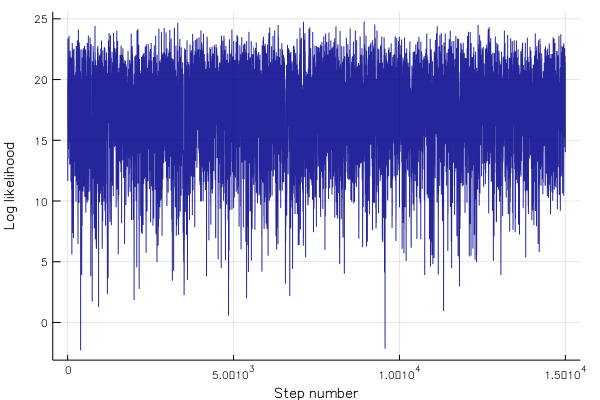## Plot results¶ In : # Plot results (mean and 95% confidence interval for both model and data) hdl = plot([mdl.Age_025CI; reverse(mdl.Age_975CI)],[mdl.Height; reverse(mdl.Height)], fill=(minimum(mdl.Height),0.5,:blue), label="model") plot!(hdl, mdl.Age, mdl.Height, linecolor=:blue, label="", fg_color_legend=:white) plot!(hdl, smpl.Age, smpl.Height, xerror=(smpl.Age-smpl.Age_025CI,smpl.Age_975CI-smpl.Age),label="data",seriestype=:scatter,color=:black) plot!(hdl, xlabel="Age ($(smpl.Age_Unit))", ylabel="Height ($(smpl.Height_Unit))") savefig(hdl,"AgeDepthModel.pdf"); display(hdl)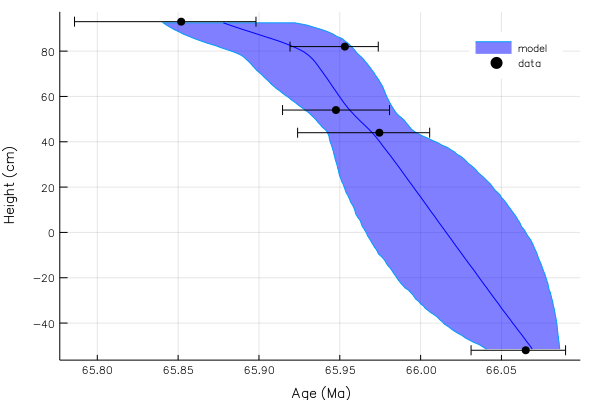In : # Interpolate results at KTB (height = 0) height = 0 KTB = linterp1s(mdl.Height,mdl.Age,height) KTB_min = linterp1s(mdl.Height,mdl.Age_025CI,height) KTB_max = linterp1s(mdl.Height,mdl.Age_975CI,height) print("Interpolated age:$KTB +$(KTB_max-KTB)/-$(KTB-KTB_min) Ma")

# We can also interpolate the full distribution:
interpolated_distribution = Array{Float64}(undef,size(agedist,2))
for i=1:size(agedist,2)
interpolated_distribution[i] = linterp1s(mdl.Height,agedist[:,i],height)
end
histogram(interpolated_distribution, xlabel="Age (Ma)", ylabel="N", label="", fill=(0.85,:darkblue), linecolor=:darkblue)

Out: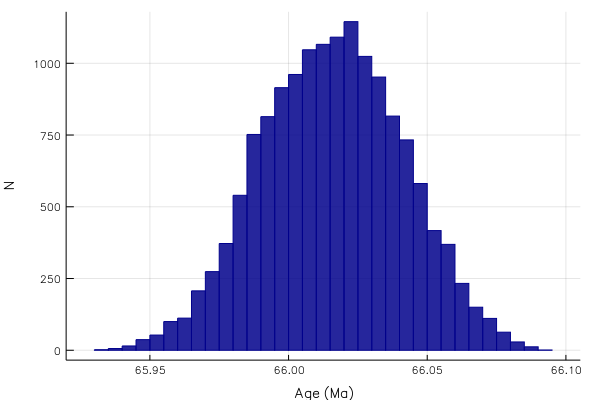Interpolated age: 66.01580546918152 +0.04924877964148777/-0.049571492234548487 Ma

There are other things we can plot as well, such as deposition rate:

In :
# Set bin width and spacing
binwidth = 0.01 # Myr
binoverlap = 10
ages = collect(minimum(mdl.Age):binwidth/binoverlap:maximum(mdl.Age))
bincenters = ages[1+Int(binoverlap/2):end-Int(binoverlap/2)]
spacing = binoverlap

# Calculate rates for the stratigraphy of each markov chain step
dhdt_dist = Array{Float64}(undef, length(ages)-binoverlap, config.nsteps)
@time for i=1:config.nsteps
heights = linterp1(reverse(agedist[:,i]), reverse(mdl.Height), ages)
dhdt_dist[:,i] = abs.(heights[1:end-spacing] - heights[spacing+1:end]) ./ binwidth
end

# Find mean and 1-sigma (68%) CI
dhdt = nanmean(dhdt_dist,dim=2)
dhdt_50p = nanmedian(dhdt_dist,dim=2)
dhdt_16p = pctile(dhdt_dist,15.865,dim=2) # Minus 1-sigma (15.865th percentile)
dhdt_84p = pctile(dhdt_dist,84.135,dim=2) # Plus 1-sigma (84.135th percentile)
# Other confidence intervals (10:10:50)
dhdt_20p = pctile(dhdt_dist,20,dim=2)
dhdt_80p = pctile(dhdt_dist,80,dim=2)
dhdt_25p = pctile(dhdt_dist,25,dim=2)
dhdt_75p = pctile(dhdt_dist,75,dim=2)
dhdt_30p = pctile(dhdt_dist,30,dim=2)
dhdt_70p = pctile(dhdt_dist,70,dim=2)
dhdt_35p = pctile(dhdt_dist,35,dim=2)
dhdt_65p = pctile(dhdt_dist,65,dim=2)
dhdt_40p = pctile(dhdt_dist,40,dim=2)
dhdt_60p = pctile(dhdt_dist,60,dim=2)
dhdt_45p = pctile(dhdt_dist,45,dim=2)
dhdt_55p = pctile(dhdt_dist,55,dim=2)

# Plot results
hdl = plot(bincenters,dhdt, label="Mean", color=:black, linewidth=2)
plot!(hdl,[bincenters; reverse(bincenters)],[dhdt_16p; reverse(dhdt_84p)], fill=(minimum(mdl.Height),0.2,:darkblue), linealpha=0, label="68% CI")
plot!(hdl,[bincenters; reverse(bincenters)],[dhdt_20p; reverse(dhdt_80p)], fill=(minimum(mdl.Height),0.2,:darkblue), linealpha=0, label="")
plot!(hdl,[bincenters; reverse(bincenters)],[dhdt_25p; reverse(dhdt_75p)], fill=(minimum(mdl.Height),0.2,:darkblue), linealpha=0, label="")
plot!(hdl,[bincenters; reverse(bincenters)],[dhdt_30p; reverse(dhdt_70p)], fill=(minimum(mdl.Height),0.2,:darkblue), linealpha=0, label="")
plot!(hdl,[bincenters; reverse(bincenters)],[dhdt_35p; reverse(dhdt_65p)], fill=(minimum(mdl.Height),0.2,:darkblue), linealpha=0, label="")
plot!(hdl,[bincenters; reverse(bincenters)],[dhdt_40p; reverse(dhdt_60p)], fill=(minimum(mdl.Height),0.2,:darkblue), linealpha=0, label="")
plot!(hdl,[bincenters; reverse(bincenters)],[dhdt_45p; reverse(dhdt_55p)], fill=(minimum(mdl.Height),0.2,:darkblue), linealpha=0, label="")
plot!(hdl,bincenters,dhdt_50p, label="Median", color=:grey, linewidth=1)
plot!(hdl, xlabel="Age ($(smpl.Age_Unit))", ylabel="Depositional Rate ($(smpl.Height_Unit) / $(smpl.Age_Unit) over$binwidth \$(smpl.Age_Unit))", fg_color_legend=:white)
# savefig(hdl,"DepositionRateModelCI.pdf")
display(hdl)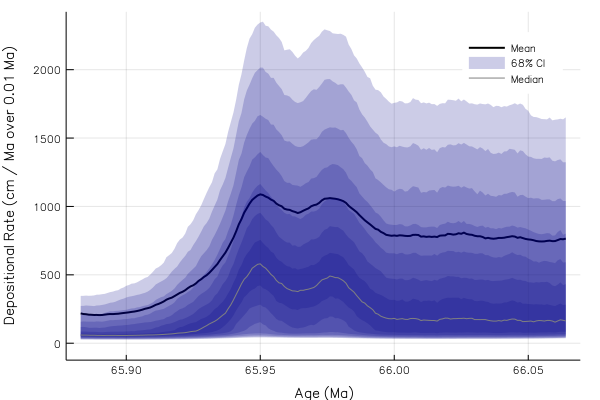## Stratigraphic model including hiatuses¶

We can also deal with discrete hiatuses in the stratigraphic section if we know where they are and about how long they lasted. We need some different models and methods though. In particular, in addition to the ChronAgeData struct, we also need a HiatusData struct now, and we're going to want to pass these to StratMetropolisDistHiatus instead of StratMetropolisDist like before.

In :
# Data about hiatuses
nHiatuses = 2 # The number of hiatuses you have data for
hiatus = NewHiatusData(nHiatuses) # Struct to hold data
hiatus.Height         = [20.0, 35.0 ]
hiatus.Height_sigma   = [ 0.0,  0.0 ]
hiatus.Duration       = [ 0.2,  0.43]
hiatus.Duration_sigma = [ 0.05, 0.07]

# Run the model. Note: we're using StratMetropolisDistHiatus now, instead of just StratMetropolisDistHiatus
(mdl, agedist, hiatusdist, lldist) = StratMetropolisDistHiatus(smpl, hiatus, config); sleep(0.5)

# Plot results (mean and 95% confidence interval for both model and data)
hdl = plot([mdl.Age_025CI; reverse(mdl.Age_975CI)],[mdl.Height; reverse(mdl.Height)], fill=(minimum(mdl.Height),0.5,:blue), label="model")
plot!(hdl, mdl.Age, mdl.Height, linecolor=:blue, label="", fg_color_legend=:white)
plot!(hdl, smpl.Age, smpl.Height, xerror=(smpl.Age-smpl.Age_025CI,smpl.Age_975CI-smpl.Age),label="data",seriestype=:scatter,color=:black)
plot!(hdl, xlabel="Age (Ma)", ylabel="Height (cm)")

  0.909426 seconds (707.88 k allocations: 231.491 MiB, 31.11% gc time)
Generating stratigraphic age-depth model...
Burn-in: 1750000 steps

Burn-in...100%|█████████████████████████████████████████|  ETA: 0:00:04
Collecting sieved stationary distribution: 2625000 steps

Burn-in...100%|█████████████████████████████████████████| Time: 0:18:05
Collecting...100%|██████████████████████████████████████| Time: 0:23:32

Out: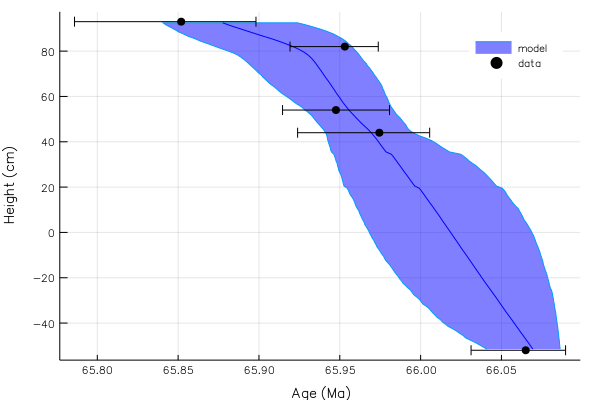In [ ]: